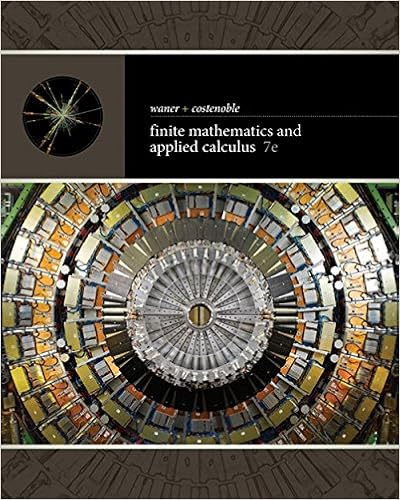# hwk-6-mfe-2 - Math for Econ II Written Assignment 6(28...

• Test Prep
• xuanlinh95
• 1

This preview shows page 1 out of 1 page.

##### We have textbook solutions for you!
The document you are viewing contains questions related to this textbook.The document you are viewing contains questions related to this textbook.
Chapter 5 / Exercise 2
Finite Mathematics and Applied Calculus
Costenoble/WanerExpert Verified
Math for Econ II, Written Assignment 6 (28 points)Due Friday, October 30Please write neat solutions for the problems below. Show all your work. If you only write the answer with no work,you will not be given any credit.Write your name and recitation section number.Staple your homework if you have multiple pages!1. (3 pts) Find all numbersxsuch thatx0-112x-13-201= 5.2. (4 pts) Find123410-124200400-3-6-9-12400111.3. (4 pts) There are 2×2 matricesAsuch thatA2=-I2. For example, ifA=0-110, thenA2=-I2.Is there a 3×3 matrixAsuch thatA2=-I3? If so, find such anA. If not, show no suchAexists.(hint: use the fact that ifXandYaren×nmatrices then|XY|=|X||Y|)4. Solve the following systems by finding the inverse of the coefficient matrix.(a) (2 pts)
##### We have textbook solutions for you!
The document you are viewing contains questions related to this textbook.The document you are viewing contains questions related to this textbook.
Chapter 5 / Exercise 2
Finite Mathematics and Applied Calculus
Costenoble/WanerExpert Verified
•••